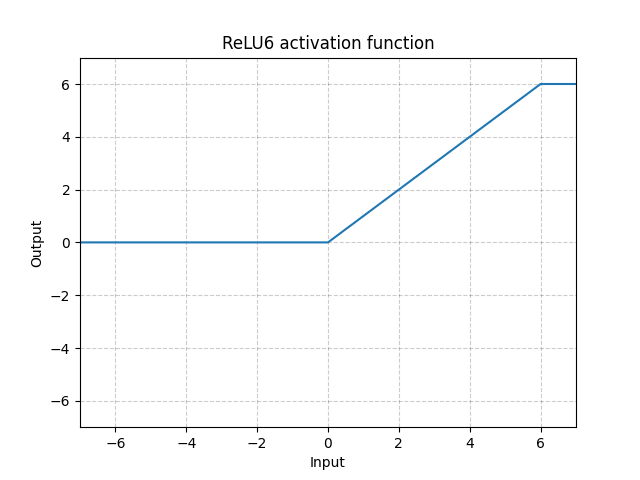/PyTorch

# ReLU6

class torch.nn.ReLU6(inplace: bool = False) [source]

Applies the element-wise function:

$\text{ReLU6}(x) = \min(\max(0,x), 6)$
Parameters

inplace – can optionally do the operation in-place. Default: False

Shape:
• Input: $(N, *)$ where * means, any number of additional dimensions
• Output: $(N, *)$ , same shape as the inputExamples:

>>> m = nn.ReLU6()
>>> input = torch.randn(2)
>>> output = m(input)


© 2019 Torch Contributors
Licensed under the 3-clause BSD License.
https://pytorch.org/docs/1.7.0/generated/torch.nn.ReLU6.html